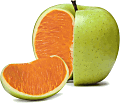## OT: Bias of AUCs; example [Regulatives / Guidelines]

Massa,
I dunno understand wat ya sayin’.❝ there is no doubt that AUC calculation can be biased.

OK.

❝ Whether the ln difference or ratio is biased, generally, is another matter and this is where I meant to draw the attention - this is the BE outcome. If one could make a case for saying that this bias affects T more often or to a larger extent than it affects R, generally, then that is where the use of this approach would be not just ugly but even worse also clearly biased.

If we can measure all concentrations, any of the methods is unbiased as is the T/R estimate (yep, even by the linear trapezoidal and the bloody AUCall). I’m only talking about cases where we deal with BQLs.

If T = R (and for nitpickers: we have balanced sequences / equal group sizes) both treatments will be affected to the same extent, the bias of the AUC will mean out and the estimate will tell used T ≈ R.
If T < R, T will be more often affected than R. If T > R, R will be more often affected than T. In the first case the T/R estimate will have a negative bias (since we have more cases where T is underestimated) and in the second a positive one (since we have more cases where R is underestimated).

Example: One-compartment, D 100, V 1, t½a 1 h, t½e 4 h, FR 1, FT 0.8–1, t 0, 1, 2, 2.5, 3, 3.5, 4, 4.5, 6, 9, 12, 16, 24; λz estimated from the last two measurable concentrations. LLOQ 3% of theoretical Cref,max (62.996). Lin-up / log-down trapezoidal method for AUC0–t, AUC0–∞, and AUC0–t(common).As long as we can measure all concentrations of both T and R, the PE of AUC0–t is unbiased. Once we drop below the LLOQ, the PE will be negatively biased because we are comparing AUC0–16 of T with AUC0–24 of R. Here it happens below a true f of 0.9071 where Clast of T with 1.8898 will be below the LLOQ of 1.8899.
The PEs of AUC0–∞ and AUC0–t(common) are always unbiased.*

Dif-tor heh smusma 🖖🏼 Довге життя Україна!Helmut SchützThe quality of responses received is directly proportional to the quality of the question asked. 🚮
Science QuotesIng. Helmut Schütz